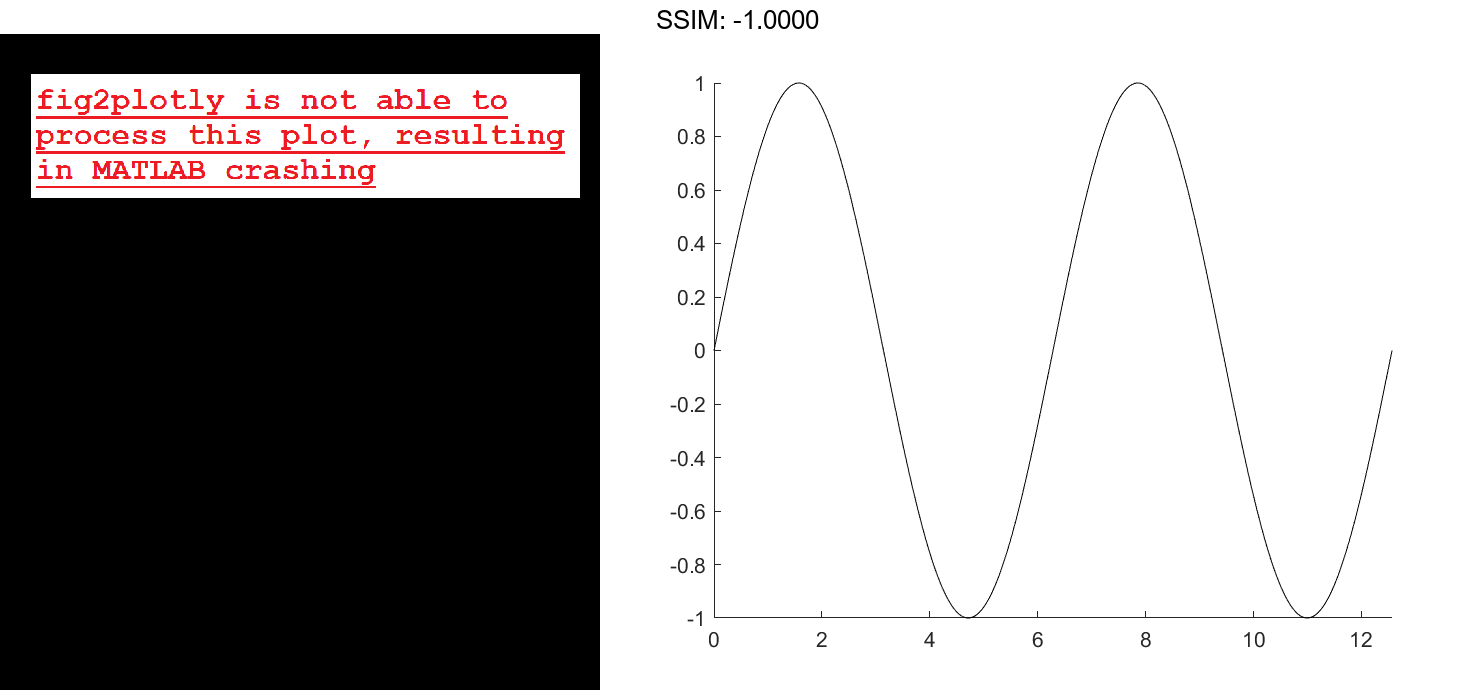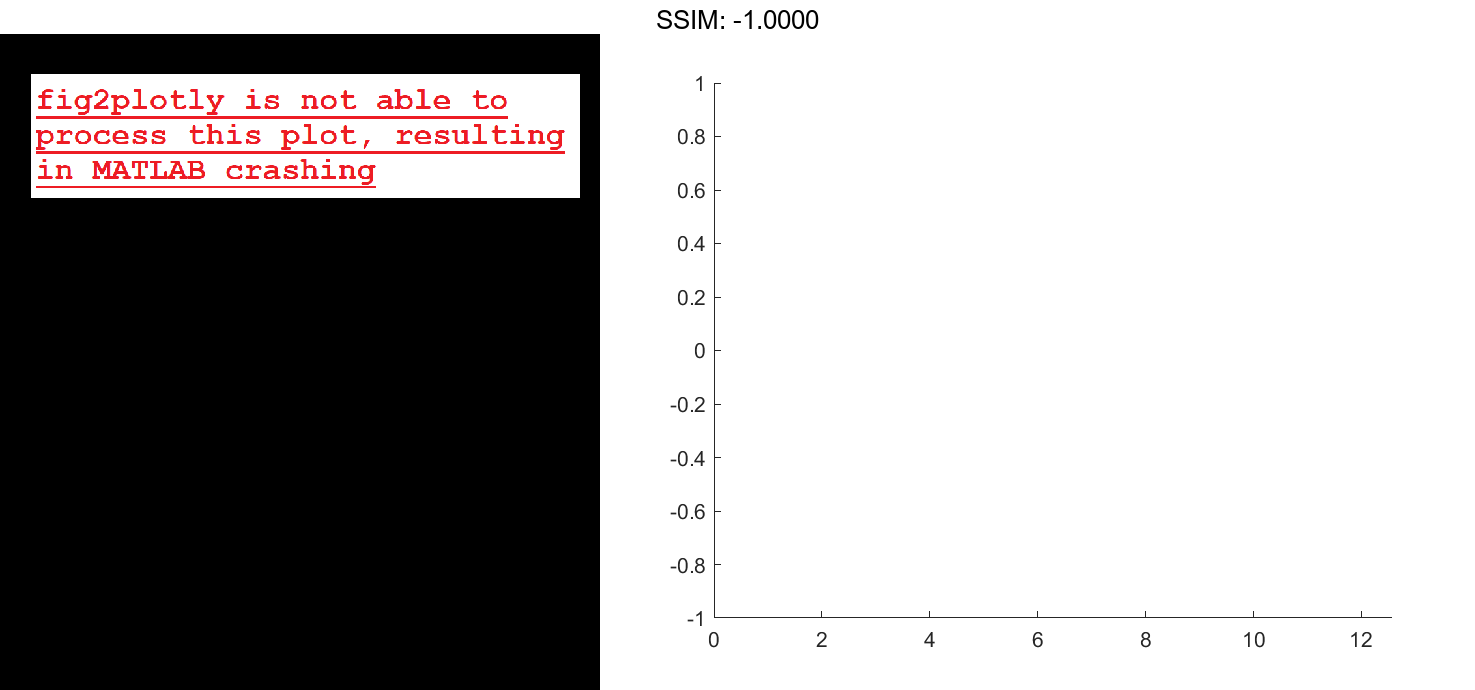# Animated Line in MATLAB®

How to make Animated Line and Comet plots in MATLAB® with Plotly.

## Display Line Animation

Create the initial animated line object. Then, use a loop to add 1,000 points to the line. After adding each new point, use drawnow to display the new point on the screen.

h = animatedline;
axis([0,4*pi,-1,1])

x = linspace(0,4*pi,1000);
y = sin(x);
for k = 1:length(x)
drawnow
end

fig2plotly()For faster rendering, add more than one point to the line each time through the loop or use drawnow limitrate.

Query the points of the line.

[xdata,ydata] = getpoints(h);

fig2plotly()


Clear the points from the line.

clearpoints(h)
drawnow

fig2plotly()## Specify Animated Line Color

Set the color of the animated line to red and set its line width to 3 points.

x = [1 2];
y = [1 2];
h = animatedline(x,y,'Color','r','LineWidth',3);

fig2plotly()## Set Maximum Number of Points

Limit the number of points in the animated line to 100. Use a loop to add one point to the line at a time. When the line contains 100 points, adding a new point to the line deletes the oldest point.

h = animatedline('MaximumNumPoints',100);
axis([0,4*pi,-1,1])

x = linspace(0,4*pi,1000);
y = sin(x);
for k = 1:length(x)